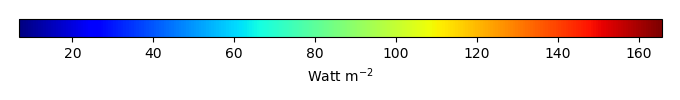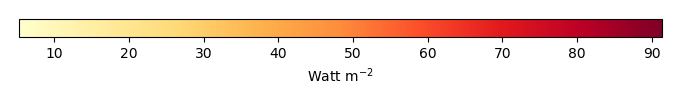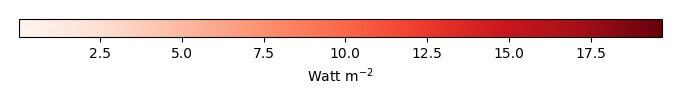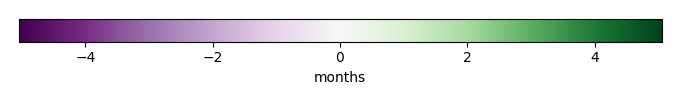# Mean State

Period Mean (original grids) [Watt m-2]
Model Period Mean (intersection) [Watt m-2]
Model Period Mean (complement) [Watt m-2]
Benchmark Period Mean (intersection) [Watt m-2]
Benchmark Period Mean (complement) [Watt m-2]
Bias [Watt m-2]
RMSE [Watt m-2]
Phase Shift [months]
Bias Score 
RMSE Score 
Seasonal Cycle Score 
Spatial Distribution Score 
Interannual Variability Score 
Overall Score 
Benchmark [-] 38.3
CRUNCEPv7 [-] 35.9 35.5 0.00 39.6 15.5 -3.45 11.0 1.68 0.35 0.38 0.73 0.94 0.44 0.54
GSWP3v1 [-] 33.8 33.5 0.00 39.6 15.5 -5.43 11.5 1.57 0.30 0.38 0.75 0.97 0.51 0.55
WFDEI [-] 31.5 31.2 0.00 39.6 15.5 -7.79 13.9 2.18 0.23 0.33 0.63 0.94 0.50 0.50
Period Mean (original grids) [Watt m-2]
Model Period Mean (intersection) [Watt m-2]
Model Period Mean (complement) [Watt m-2]
Benchmark Period Mean (intersection) [Watt m-2]
Benchmark Period Mean (complement) [Watt m-2]
Bias [Watt m-2]
RMSE [Watt m-2]
Phase Shift [months]
Bias Score 
RMSE Score 
Seasonal Cycle Score 
Spatial Distribution Score 
Interannual Variability Score 
Overall Score 
Benchmark [-] 52.3
CRUNCEPv7 [-] 47.2 47.7 0.00 52.8 14.1 -5.16 13.2 1.29 0.44 0.40 0.84 0.99 0.47 0.59
GSWP3v1 [-] 45.9 46.4 0.00 52.8 14.1 -6.56 13.5 1.32 0.40 0.38 0.84 0.99 0.50 0.58
WFDEI [-] 46.0 46.6 0.00 52.8 14.1 -6.27 15.4 1.39 0.39 0.33 0.83 0.97 0.49 0.56
Period Mean (original grids) [Watt m-2]
Model Period Mean (intersection) [Watt m-2]
Model Period Mean (complement) [Watt m-2]
Benchmark Period Mean (intersection) [Watt m-2]
Benchmark Period Mean (complement) [Watt m-2]
Bias [Watt m-2]
RMSE [Watt m-2]
Phase Shift [months]
Bias Score 
RMSE Score 
Seasonal Cycle Score 
Spatial Distribution Score 
Interannual Variability Score 
Overall Score 
Benchmark [-] 39.3
CRUNCEPv7 [-] 36.1 36.1 0.00 40.4 16.6 -4.22 12.0 1.79 0.37 0.42 0.73 0.92 0.48 0.56
GSWP3v1 [-] 36.1 36.1 0.00 40.4 16.6 -4.29 12.1 1.88 0.37 0.40 0.71 0.92 0.53 0.55
WFDEI [-] 36.4 36.4 0.00 40.4 16.6 -3.94 12.5 1.99 0.37 0.37 0.70 0.97 0.54 0.55
Period Mean (original grids) [Watt m-2]
Model Period Mean (intersection) [Watt m-2]
Model Period Mean (complement) [Watt m-2]
Benchmark Period Mean (intersection) [Watt m-2]
Benchmark Period Mean (complement) [Watt m-2]
Bias [Watt m-2]
RMSE [Watt m-2]
Phase Shift [months]
Bias Score 
RMSE Score 
Seasonal Cycle Score 
Spatial Distribution Score 
Interannual Variability Score 
Overall Score 
Benchmark [-] 31.6
CRUNCEPv7 [-] 39.6 39.5 0.00 31.5 32.6 9.02 22.2 0.253 0.73 0.54 0.98 0.98 0.76 0.76
GSWP3v1 [-] 37.2 37.2 0.00 31.5 32.6 6.18 20.2 0.280 0.77 0.55 0.98 0.87 0.80 0.75
WFDEI [-] 41.4 41.3 0.00 31.5 32.6 10.4 27.4 0.210 0.69 0.46 0.99 0.98 0.77 0.72
Period Mean (original grids) [Watt m-2]
Model Period Mean (intersection) [Watt m-2]
Model Period Mean (complement) [Watt m-2]
Benchmark Period Mean (intersection) [Watt m-2]
Benchmark Period Mean (complement) [Watt m-2]
Bias [Watt m-2]
RMSE [Watt m-2]
Phase Shift [months]
Bias Score 
RMSE Score 
Seasonal Cycle Score 
Spatial Distribution Score 
Interannual Variability Score 
Overall Score 
Benchmark [-] 23.5
CRUNCEPv7 [-] 28.5 28.5 0.00 25.9 15.5 3.10 10.9 2.16 0.40 0.38 0.66 0.20 0.42 0.41
GSWP3v1 [-] 26.9 26.9 0.00 25.9 15.5 1.64 11.1 1.56 0.34 0.37 0.77 0.22 0.54 0.43
WFDEI [-] 24.9 24.9 0.00 25.9 15.5 -0.337 11.3 1.68 0.29 0.36 0.75 0.35 0.50 0.43
Period Mean (original grids) [Watt m-2]
Model Period Mean (intersection) [Watt m-2]
Model Period Mean (complement) [Watt m-2]
Benchmark Period Mean (intersection) [Watt m-2]
Benchmark Period Mean (complement) [Watt m-2]
Bias [Watt m-2]
RMSE [Watt m-2]
Phase Shift [months]
Bias Score 
RMSE Score 
Seasonal Cycle Score 
Spatial Distribution Score 
Interannual Variability Score 
Overall Score 
Benchmark [-] 21.4
CRUNCEPv7 [-] 25.2 25.0 0.00 21.5 17.6 3.49 10.2 0.512 0.75 0.54 0.94 0.88 0.67 0.72
GSWP3v1 [-] 23.8 23.7 0.00 21.5 17.6 2.19 10.4 0.647 0.79 0.51 0.92 0.92 0.72 0.73
WFDEI [-] 24.1 23.9 0.00 21.5 17.6 2.44 11.9 0.496 0.76 0.47 0.94 0.80 0.70 0.69
Period Mean (original grids) [Watt m-2]
Model Period Mean (intersection) [Watt m-2]
Model Period Mean (complement) [Watt m-2]
Benchmark Period Mean (intersection) [Watt m-2]
Benchmark Period Mean (complement) [Watt m-2]
Bias [Watt m-2]
RMSE [Watt m-2]
Phase Shift [months]
Bias Score 
RMSE Score 
Seasonal Cycle Score 
Spatial Distribution Score 
Interannual Variability Score 
Overall Score 
Benchmark [-] 30.0
CRUNCEPv7 [-] 38.7 38.6 0.00 30.5 14.9 7.41 13.8 1.05 0.56 0.44 0.86 0.95 0.64 0.65
GSWP3v1 [-] 35.0 34.9 0.00 30.5 14.9 3.75 11.5 0.980 0.67 0.47 0.87 0.96 0.67 0.68
WFDEI [-] 38.4 38.3 0.00 30.5 14.9 7.00 16.2 1.20 0.58 0.38 0.83 0.86 0.68 0.62
Period Mean (original grids) [Watt m-2]
Model Period Mean (intersection) [Watt m-2]
Model Period Mean (complement) [Watt m-2]
Benchmark Period Mean (intersection) [Watt m-2]
Benchmark Period Mean (complement) [Watt m-2]
Bias [Watt m-2]
RMSE [Watt m-2]
Phase Shift [months]
Bias Score 
RMSE Score 
Seasonal Cycle Score 
Spatial Distribution Score 
Interannual Variability Score 
Overall Score 
Benchmark [-] 29.5
CRUNCEPv7 [-] 36.6 36.6 0.00 30.6 14.2 5.15 12.0 0.893 0.56 0.41 0.90 0.97 0.46 0.62
GSWP3v1 [-] 32.7 32.7 0.00 30.6 14.2 1.54 11.6 0.981 0.57 0.40 0.89 0.98 0.50 0.62
WFDEI [-] 32.6 32.6 0.00 30.6 14.2 1.33 12.8 1.06 0.53 0.36 0.88 0.93 0.50 0.59
Period Mean (original grids) [Watt m-2]
Model Period Mean (intersection) [Watt m-2]
Model Period Mean (complement) [Watt m-2]
Benchmark Period Mean (intersection) [Watt m-2]
Benchmark Period Mean (complement) [Watt m-2]
Bias [Watt m-2]
RMSE [Watt m-2]
Phase Shift [months]
Bias Score 
RMSE Score 
Seasonal Cycle Score 
Spatial Distribution Score 
Interannual Variability Score 
Overall Score 
Benchmark [-] 33.0
CRUNCEPv7 [-] 39.6 39.4 0.00 32.8 37.1 7.10 22.6 0.279 0.78 0.56 0.98 0.96 0.69 0.75
GSWP3v1 [-] 35.8 35.6 0.00 32.8 37.1 3.16 18.9 0.279 0.83 0.58 0.98 0.82 0.76 0.76
WFDEI [-] 38.9 38.6 0.00 32.8 37.1 6.21 25.4 0.167 0.79 0.49 0.99 0.93 0.72 0.74
Period Mean (original grids) [Watt m-2]
Model Period Mean (intersection) [Watt m-2]
Model Period Mean (complement) [Watt m-2]
Benchmark Period Mean (intersection) [Watt m-2]
Benchmark Period Mean (complement) [Watt m-2]
Bias [Watt m-2]
RMSE [Watt m-2]
Phase Shift [months]
Bias Score 
RMSE Score 
Seasonal Cycle Score 
Spatial Distribution Score 
Interannual Variability Score 
Overall Score 
Benchmark [-] 35.1
CRUNCEPv7 [-] 42.4 42.4 0.00 36.7 14.1 4.32 10.8 0.616 0.57 0.55 0.95 0.97 0.62 0.70
GSWP3v1 [-] 41.4 41.5 0.00 36.7 14.1 3.44 10.5 0.452 0.59 0.54 0.96 0.96 0.64 0.71
WFDEI [-] 42.5 42.6 0.00 36.7 14.1 4.40 11.6 0.586 0.58 0.49 0.95 0.98 0.71 0.70
Period Mean (original grids) [Watt m-2]
Model Period Mean (intersection) [Watt m-2]
Model Period Mean (complement) [Watt m-2]
Benchmark Period Mean (intersection) [Watt m-2]
Benchmark Period Mean (complement) [Watt m-2]
Bias [Watt m-2]
RMSE [Watt m-2]
Phase Shift [months]
Bias Score 
RMSE Score 
Seasonal Cycle Score 
Spatial Distribution Score 
Interannual Variability Score 
Overall Score 
Benchmark [-] 19.8
CRUNCEPv7 [-] 25.8 25.8 0.00 20.7 10.0 4.62 10.7 0.995 0.65 0.54 0.89 0.96 0.65 0.70
GSWP3v1 [-] 21.6 21.6 0.00 20.7 10.0 0.867 9.68 1.06 0.72 0.54 0.88 0.83 0.65 0.69
WFDEI [-] 23.4 23.4 0.00 20.7 10.0 2.45 11.6 1.38 0.70 0.48 0.81 0.96 0.68 0.68
Period Mean (original grids) [Watt m-2]
Model Period Mean (intersection) [Watt m-2]
Model Period Mean (complement) [Watt m-2]
Benchmark Period Mean (intersection) [Watt m-2]
Benchmark Period Mean (complement) [Watt m-2]
Bias [Watt m-2]
RMSE [Watt m-2]
Phase Shift [months]
Bias Score 
RMSE Score 
Seasonal Cycle Score 
Spatial Distribution Score 
Interannual Variability Score 
Overall Score 
Benchmark [-] 23.6
CRUNCEPv7 [-] 47.5 47.5 0.00 43.1 14.1 3.57 16.6 0.876 0.59 0.49 0.89 0.88 0.54 0.65
GSWP3v1 [-] 44.9 44.9 0.00 43.1 14.1 0.998 14.3 0.866 0.63 0.50 0.89 0.88 0.59 0.66
WFDEI [-] 47.5 47.5 0.00 43.1 14.1 3.63 18.7 0.981 0.57 0.44 0.87 0.89 0.61 0.64
Period Mean (original grids) [Watt m-2]
Model Period Mean (intersection) [Watt m-2]
Model Period Mean (complement) [Watt m-2]
Benchmark Period Mean (intersection) [Watt m-2]
Benchmark Period Mean (complement) [Watt m-2]
Bias [Watt m-2]
RMSE [Watt m-2]
Phase Shift [months]
Bias Score 
RMSE Score 
Seasonal Cycle Score 
Spatial Distribution Score 
Interannual Variability Score 
Overall Score 
Benchmark [-] 64.3
CRUNCEPv7 [-] 81.4 81.2 0.00 66.2 16.5 12.9 20.9 0.649 0.53 0.55 0.94 0.98 0.53 0.68
GSWP3v1 [-] 78.4 78.2 0.00 66.2 16.5 10.1 18.6 0.505 0.56 0.58 0.95 0.98 0.54 0.70
WFDEI [-] 82.8 82.6 0.00 66.2 16.5 14.3 22.1 0.741 0.50 0.55 0.92 0.98 0.53 0.67
Period Mean (original grids) [Watt m-2]
Model Period Mean (intersection) [Watt m-2]
Model Period Mean (complement) [Watt m-2]
Benchmark Period Mean (intersection) [Watt m-2]
Benchmark Period Mean (complement) [Watt m-2]
Bias [Watt m-2]
RMSE [Watt m-2]
Phase Shift [months]
Bias Score 
RMSE Score 
Seasonal Cycle Score 
Spatial Distribution Score 
Interannual Variability Score 
Overall Score 
Benchmark [-] 34.3
CRUNCEPv7 [-] 43.2 43.2 0.00 34.2 35.0 10.5 25.3 0.373 0.69 0.54 0.97 0.96 0.69 0.73
GSWP3v1 [-] 39.0 38.9 0.00 34.2 35.0 5.70 19.5 0.221 0.77 0.58 0.98 0.96 0.80 0.78
WFDEI [-] 44.9 44.9 0.00 34.2 35.0 12.1 30.1 0.268 0.67 0.46 0.98 0.95 0.77 0.72
Period Mean (original grids) [Watt m-2]
Model Period Mean (intersection) [Watt m-2]
Model Period Mean (complement) [Watt m-2]
Benchmark Period Mean (intersection) [Watt m-2]
Benchmark Period Mean (complement) [Watt m-2]
Bias [Watt m-2]
RMSE [Watt m-2]
Phase Shift [months]
Bias Score 
RMSE Score 
Seasonal Cycle Score 
Spatial Distribution Score 
Interannual Variability Score 
Overall Score 
Benchmark [-] 24.8
CRUNCEPv7 [-] 33.8 33.8 0.00 27.0 14.3 6.34 10.8 0.818 0.51 0.45 0.91 0.84 0.50 0.61
GSWP3v1 [-] 33.8 33.8 0.00 27.0 14.3 6.38 10.9 0.849 0.53 0.44 0.91 0.90 0.55 0.63
WFDEI [-] 34.3 34.3 0.00 27.0 14.3 6.46 11.3 0.941 0.50 0.42 0.90 0.97 0.56 0.63
Period Mean (original grids) [Watt m-2]
Model Period Mean (intersection) [Watt m-2]
Model Period Mean (complement) [Watt m-2]
Benchmark Period Mean (intersection) [Watt m-2]
Benchmark Period Mean (complement) [Watt m-2]
Bias [Watt m-2]
RMSE [Watt m-2]
Phase Shift [months]
Bias Score 
RMSE Score 
Seasonal Cycle Score 
Spatial Distribution Score 
Interannual Variability Score 
Overall Score 
Benchmark [-] 20.7
CRUNCEPv7 [-] 29.9 29.8 0.00 21.1 12.1 8.37 11.5 0.860 0.45 0.47 0.87 0.95 0.63 0.64
GSWP3v1 [-] 26.8 26.7 0.00 21.1 12.1 5.37 9.16 0.893 0.60 0.49 0.87 0.99 0.63 0.68
WFDEI [-] 31.3 31.2 0.00 21.1 12.1 9.61 14.9 1.50 0.43 0.36 0.76 0.70 0.67 0.55
Period Mean (original grids) [Watt m-2]
Model Period Mean (intersection) [Watt m-2]
Model Period Mean (complement) [Watt m-2]
Benchmark Period Mean (intersection) [Watt m-2]
Benchmark Period Mean (complement) [Watt m-2]
Bias [Watt m-2]
RMSE [Watt m-2]
Phase Shift [months]
Bias Score 
RMSE Score 
Seasonal Cycle Score 
Spatial Distribution Score 
Interannual Variability Score 
Overall Score 
Benchmark [-] 20.3
CRUNCEPv7 [-] 26.2 26.4 0.00 20.6 10.6 5.73 11.9 0.756 0.60 0.52 0.90 0.86 0.66 0.68
GSWP3v1 [-] 23.5 23.6 0.00 20.6 10.6 2.92 10.2 0.740 0.69 0.53 0.91 0.93 0.68 0.71
WFDEI [-] 25.6 25.7 0.00 20.6 10.6 5.04 13.0 0.636 0.63 0.46 0.92 0.83 0.69 0.66
Period Mean (original grids) [Watt m-2]
Model Period Mean (intersection) [Watt m-2]
Model Period Mean (complement) [Watt m-2]
Benchmark Period Mean (intersection) [Watt m-2]
Benchmark Period Mean (complement) [Watt m-2]
Bias [Watt m-2]
RMSE [Watt m-2]
Phase Shift [months]
Bias Score 
RMSE Score 
Seasonal Cycle Score 
Spatial Distribution Score 
Interannual Variability Score 
Overall Score 
Benchmark [-] 30.8
CRUNCEPv7 [-] 34.8 34.9 0.00 31.1 10.8 3.36 10.1 0.900 0.66 0.51 0.87 0.97 0.71 0.70
GSWP3v1 [-] 33.2 33.2 0.00 31.1 10.8 1.78 9.03 0.712 0.72 0.53 0.90 0.98 0.72 0.73
WFDEI [-] 39.3 39.3 0.00 31.1 10.8 7.69 14.6 1.62 0.54 0.40 0.74 0.90 0.67 0.61
Period Mean (original grids) [Watt m-2]
Model Period Mean (intersection) [Watt m-2]
Model Period Mean (complement) [Watt m-2]
Benchmark Period Mean (intersection) [Watt m-2]
Benchmark Period Mean (complement) [Watt m-2]
Bias [Watt m-2]
RMSE [Watt m-2]
Phase Shift [months]
Bias Score 
RMSE Score 
Seasonal Cycle Score 
Spatial Distribution Score 
Interannual Variability Score 
Overall Score 
Benchmark [-] 26.8
CRUNCEPv7 [-] 27.9 27.9 0.00 27.1 15.2 0.878 8.23 1.65 0.71 0.38 0.76 0.66 0.40 0.55
GSWP3v1 [-] 26.5 26.5 0.00 27.1 15.2 -0.513 8.29 2.29 0.74 0.37 0.63 0.76 0.45 0.55
WFDEI [-] 24.2 24.2 0.00 27.1 15.2 -2.80 8.89 1.93 0.61 0.36 0.70 0.87 0.42 0.56
Period Mean (original grids) [Watt m-2]
Model Period Mean (intersection) [Watt m-2]
Model Period Mean (complement) [Watt m-2]
Benchmark Period Mean (intersection) [Watt m-2]
Benchmark Period Mean (complement) [Watt m-2]
Bias [Watt m-2]
RMSE [Watt m-2]
Phase Shift [months]
Bias Score 
RMSE Score 
Seasonal Cycle Score 
Spatial Distribution Score 
Interannual Variability Score 
Overall Score 
Benchmark [-] 29.5
CRUNCEPv7 [-] 31.6 31.6 0.00 29.9 14.5 1.67 9.00 1.00 0.68 0.46 0.88 0.47 0.43 0.56
GSWP3v1 [-] 30.6 30.6 0.00 29.9 14.5 0.715 8.62 1.06 0.76 0.45 0.86 0.66 0.48 0.61
WFDEI [-] 29.2 29.2 0.00 29.9 14.5 -0.689 8.94 1.02 0.71 0.44 0.87 0.65 0.51 0.60
Period Mean (original grids) [Watt m-2]
Model Period Mean (intersection) [Watt m-2]
Model Period Mean (complement) [Watt m-2]
Benchmark Period Mean (intersection) [Watt m-2]
Benchmark Period Mean (complement) [Watt m-2]
Bias [Watt m-2]
RMSE [Watt m-2]
Phase Shift [months]
Bias Score 
RMSE Score 
Seasonal Cycle Score 
Spatial Distribution Score 
Interannual Variability Score 
Overall Score 
Benchmark [-] 28.0
CRUNCEPv7 [-] 32.7 32.8 0.00 29.7 12.6 2.72 13.6 0.870 0.49 0.48 0.91 0.75 0.54 0.61
GSWP3v1 [-] 32.0 32.0 0.00 29.7 12.6 1.93 12.1 0.835 0.56 0.49 0.91 0.92 0.59 0.66
WFDEI [-] 33.7 33.8 0.00 29.7 12.6 3.73 13.8 0.997 0.54 0.45 0.88 0.96 0.61 0.65

# Temporally integrated period mean

BENCHMARK MEANMODEL MEANBIASBIAS SCORERMSERMSE SCOREBENCHMARK INTERANNUAL VARIABILITYMODEL INTERANNUAL VARIABILITYINTERANNUAL VARIABILITY SCOREBENCHMARK MAX MONTHMODEL MAX MONTHDIFFERENCE IN MAX MONTHSEASONAL CYCLE SCORESPATIAL TAYLOR DIAGRAMMODEL COLORS# Spatially integrated regional mean

MODEL COLORSREGIONAL MEANANNUAL CYCLEMONTHLY ANOMALYANNUAL CYCLE# All Models

BenchmarkCRUNCEPv7GSWP3v1WFDEI# Data Information

creation_date: Mon Jun 30 23:28:16 PDT 2014

source_file: This product is generated from monthly 1 degree GEWEX SRB Radiation observations